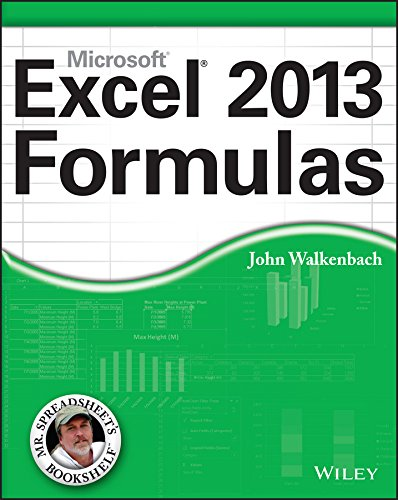# Excel 2013 Formulas

Rating: 4.2 out of 5 39 reviews
• Length: 864 pages
• Edition: 1
• Publisher:
• Publication Date: 2013-04-01
• ISBN-10: 1118490444
• ISBN-13: 9781118490440

## Book Description

Maximize the power of Excel 2013 formulas with this must-have Excel reference

John Walkenbach, known as Mr. Spreadsheet, is a master at deciphering complex technical topics and Excel formulas are no exception. This fully updated book delivers more than 800 pages of Excel 2013 tips, tricks, and techniques for creating formulas that calculate, developing custom worksheet functions with VBA, debugging formulas, and much more.

Demonstrates how to use all the latest features in Excel 2013 Shows how to create financial formulas and tap into the power of array formulas Serves as a guide to using various lookup formulas, working with conditional formatting, and developing custom functions Shares proven solutions for handling typical (and not-so-typical) Excel formula challenges Includes links to the Mr. Spreadsheet website, which contains all the templates and worksheets used in the book, plus access to John Walkenbach's award-winning Power Utility Pak. From charts to PivotTables and everything in between, Excel 2013 Formulas is your formula for Excel success.

``````PART I: Basic Information
Chapter 1: Excel in a Nutshell
Chapter 2: Basic Facts about Formulas
Chapter 3: Working with Names

PART II: Using Functions in Your Formulas
Chapter 4: Introducing Worksheet Functions
Chapter 5: Manipulating Text
Chapter 6: Working with Dates and Times
Chapter 7: Counting and Summing Techniques
Chapter 8: Using Lookup Functions
Chapter 9: Working with Tables and Lists
Chapter 10: Miscellaneous Calculations

PART III: Financial Formulas
Chapter 11: Borrowing and Investing Formulas
Chapter 12: Discounting and Depreciation Formulas
Chapter 13: Financial Schedules

PART IV: Array Formulas
Chapter 14: Introducing Arrays
Chapter 15: Performing Magic with Array Formulas

PART V: Miscellaneous Formula Techniques
Chapter 16: Importing and Cleaning Data
Chapter 17: Charting Techniques
Chapter 18: Pivot Tables
Chapter 19: Conditional Formatting
Chapter 20: Using Data Validation
Chapter 21: Creating Megaformulas
Chapter 22: Tools and Methods for Debugging Formulas

PART VI: Developing Custom Worksheet Functions
Chapter 23: Introducing VBA
Chapter 24: Function Procedure Basics
Chapter 25: VBA Programming Concepts
Chapter 26: VBA Custom Function Examples

PART VII: Appendixes
Appendix A: Excel Function Reference
Appendix B: Using Custom Number Formats``````